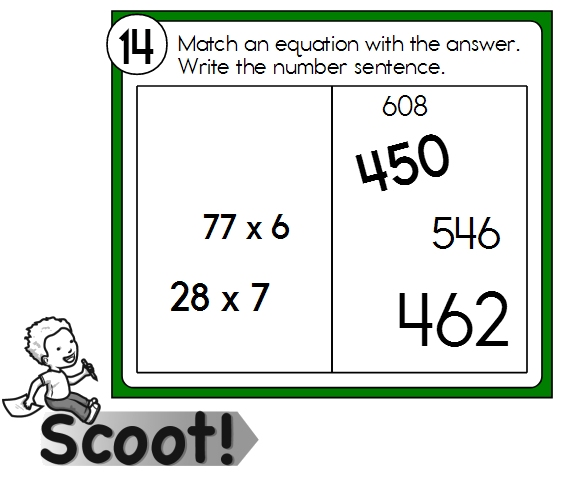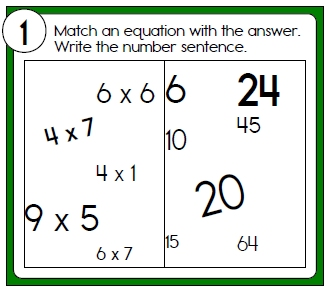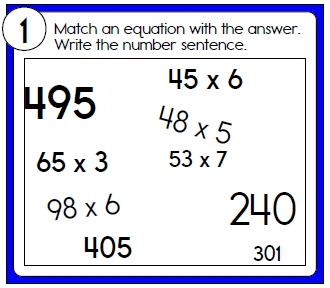Worksheets and No Prep Teaching Resources
Scoot Games
Scoot Math

# Scoot Multiplication Math GamesMultiplication Scoot Games - Products on right of card1-Digit Multiplication 1-Digit Multiplication: Easier (fewer on card) 1-Digit Multiplication 1-Digit and 2-Digit Multiplication 1-Digit and 2-Digit Multiplication: Easier (fewer on card) 1-Digit and 2-Digit Multiplication

Multiplication Scoot Games - Mixed up (little more challenging; but fun!)1-Digit Multiplication 1-Digit Multiplication: Easier (fewer on card) 1-Digit Multiplication 1-Digit and 2-Digit Multiplication 1-Digit and 2-Digit Multiplication: Easier (fewer on card) 1-Digit and 2-Digit Multiplication